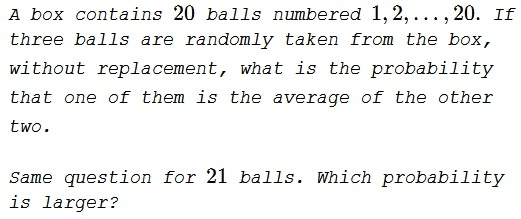# Numbered Balls Out Of a Box

### Problem### Solution 1

The average of two integers is an integer, if the two summands are of the same parity: either both are odd or both are even. These two are the largest and the smallest of the three while the middle one is uniquely determined by these two.

The number of ways to choose two even number out of $20$ is $\displaystyle {10\choose 2}=45.$ Similarly, there are $45$ odd pairs.

The total number of possible selection of three balls out of twenty is $\displaystyle {20\choose 3}=1140,$ giving the required probability as

$\displaystyle \frac{45+45}{1140}=\frac{9}{114}=\frac{3}{38}.$

If there are $21$ ball, then the corresponding probability is

$\displaystyle \frac{10\choose 2}+{11\choose 2}}{21\choose 3}}= \frac{45+55}{1330}=\frac{10}{133}\lt\frac{3}{38}$

### Solution 2

Let the total number of balls be $N$. Out of the three numbers chosen, let $i$ be the middle number. If the smallest number is $i-k$, then the largest number has to be $i+k$. Thus, for the a fixed $i$, the number of ways of choosing the other pair of numbers is equal to the number of ways of choosing a valid $k$ and is given by $min\{i-1,N-i\}$. Thus, the number of ways of choosing the balls satisfying the constrains is

\displaystyle \begin{align} N_c&=\sum_{i=1}^N min\{i-1,N-i\} \\ &=\sum_{i=1}^{\lceil \frac{N}{2}\rceil} (i-1)+\sum_{i=1+\lceil \frac{N}{2} \rceil}^N (N-i) \end{align}

Thus, the required probabilities are

\displaystyle \begin{align} P(20)&=\frac{\sum_{i=1}^{10} (i-1)+\sum_{i=11}^{20} (20-i)}{C(20,3)}=\frac{45+45}{1140}=\frac{3}{38} \\ P(21)&=\frac{\sum_{i=1}^{11} (i-1)+\sum_{i=12}^{21} (21-i)}{C(21,3)}= \frac{55+45}{1330}=\frac{10}{133}. \end{align}

$P(20)>P(21)$.

### Acknowledgment

The problem and Solution 1 are from the reference below. Solution 2 is by Amit Itagi.

### References

1. J. G. McLoughlin et all, Jim Totten's Problems of the Week, World Scientific, 2013, problem #320.